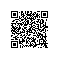# HDOJ(HDU) 2520 我是菜鸟，我怕谁(等差数列)

Problem Description
lin2144是一只小菜鸟，都是笨鸟先飞，lin2144想来个菜鸟先飞，他从0点出发

Input

Output

Sample Input
2
1
2

Sample Output
1
4

import java.util.Scanner;

public class Main{
static int db[] = new int;
public static void main(String[] args) {
dabiao();
Scanner sc = new Scanner(System.in);
int t =sc.nextInt();
while(t-->0){
int n =sc.nextInt();
System.out.println(db[n]);
}
}
private static void dabiao() {
db=1;
int sum=1;
for(int i=2;i<=100000;i++){
sum=(sum+2)%10000;
db[i]=(db[i-1]+sum)%10000;
}
}
}使用钉钉扫一扫加入圈子
+ 订阅